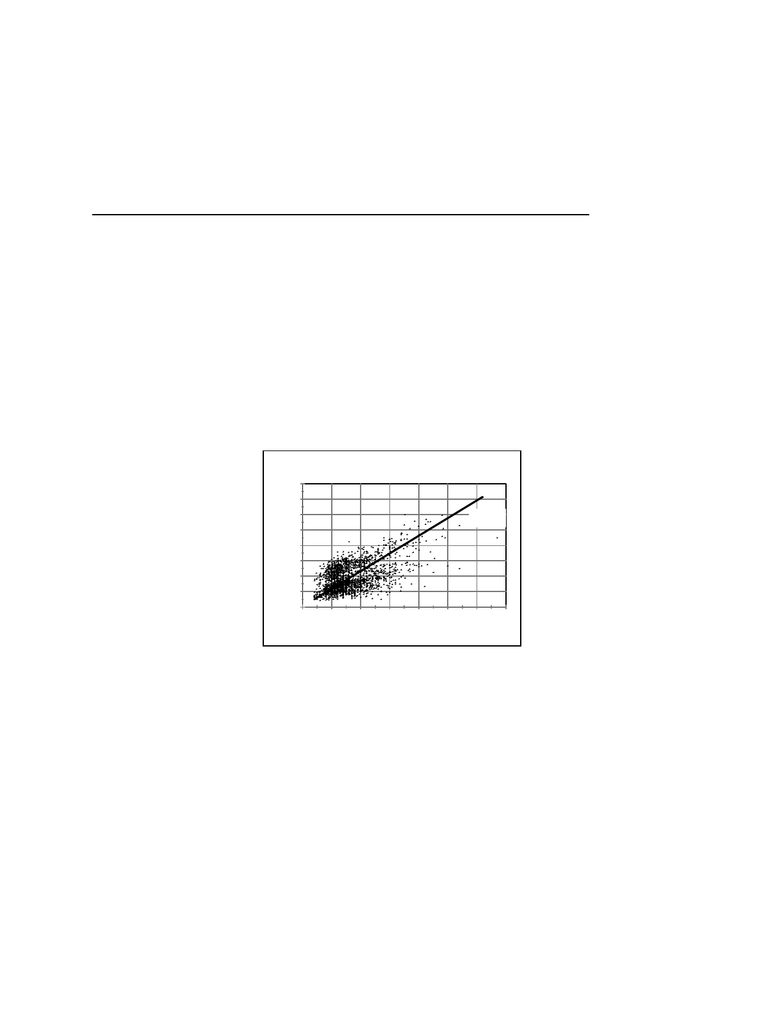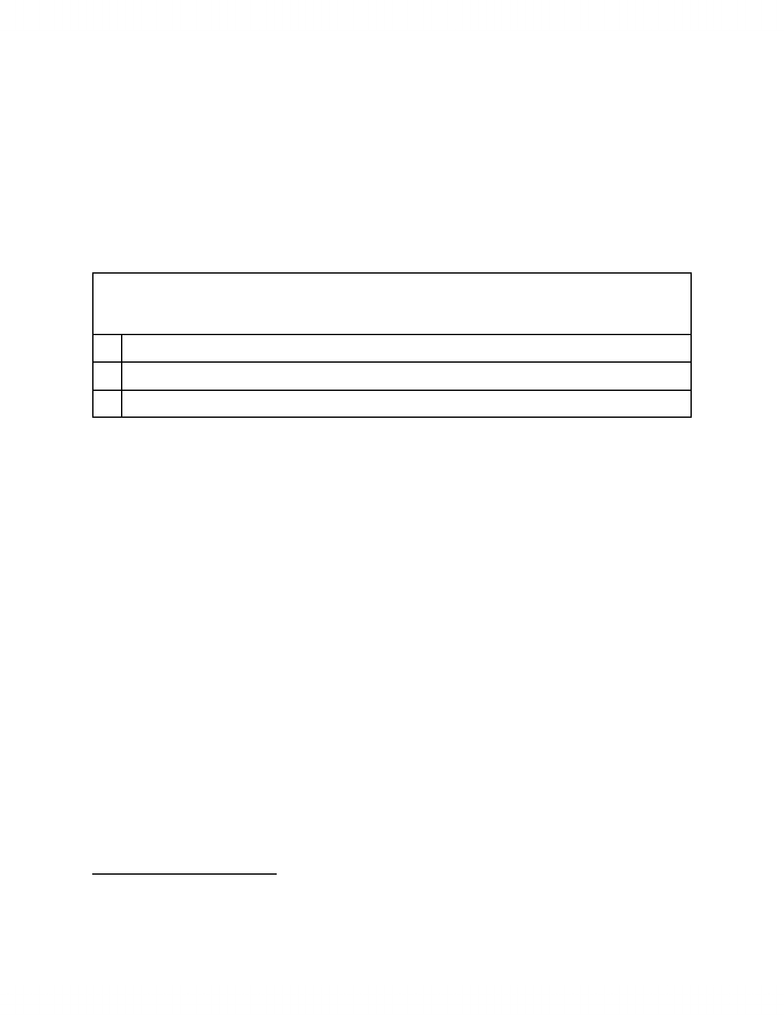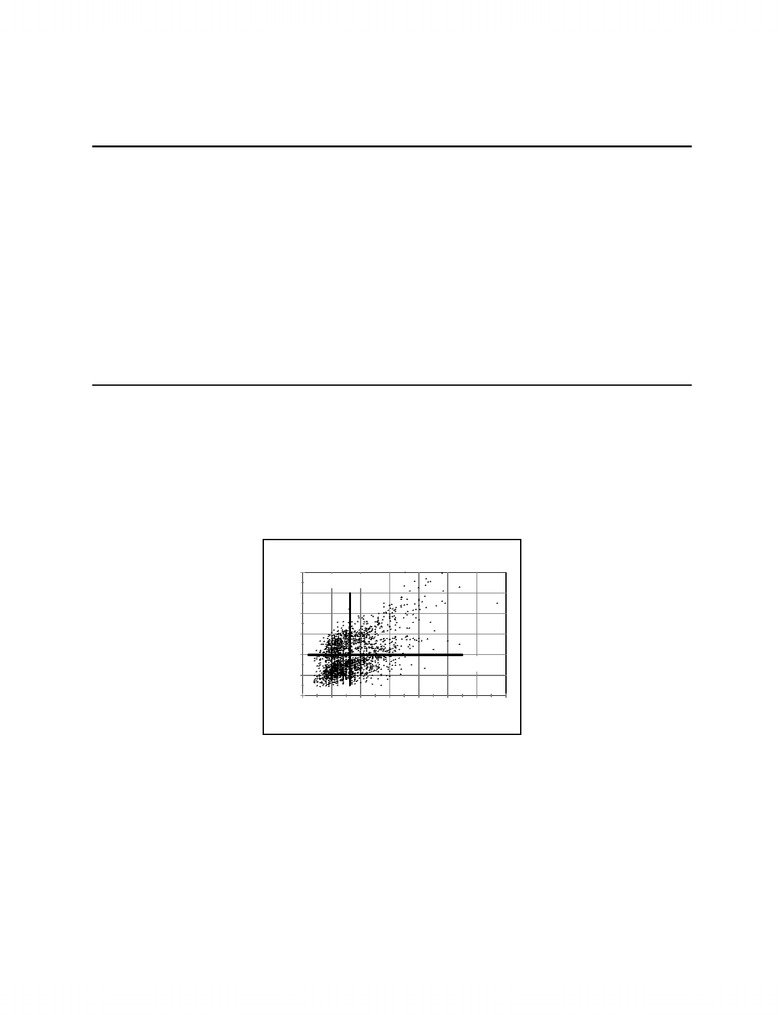# Ch2.pdf

39 views30 pages
School
Department
Course0
50
100
150
200
250
300
350
400
Thousands
500 1000 1500 2000 2500 3000 3500 4000
Size (square feet)
House Prices Vrs Size
1983-86 & 1987-88 Figure 2.1
Price
Major
Axis
Chapter 2
Bivariate Distributions
2.1 Descriptive Statistics for Bivariate Distributions: Covariance and Correlation
Chapter 1 focuses on the distribution of single random variables, such as the wage received by an
individual worker. Empirical studies in economics typically seek to understand the relationship between
two or more random variables. For example, how does the level of education affect the wage a worker
can expect to earn? This chapter considers bivariate distributions; the statistical relationship between a
pair of random variables. For example, if X and Y are both normally distributed random variables, their
joint distribution is known as the bivariate normal distribution. Before exploring the properties of a
theoretical distribution such as the bivariate normal, we will consider how descriptive statistics can be
used to capture some essential features of the relationship between a pair of variables.
Figure 2.1 is a scatter diagram of house prices and house sizes. Each point in the diagram
corresponds to the price and size of a particular house. The sample consists of 2181 observations on
house sales gathered over a 6 year period: 1983-88 (but the sample has no data for 1986.) Price refers to
the price for which the house sold and size is the total floor space of the house measured in square feet.
The scatter plot reveals a positive relationship between size and price - the scatter of points stretches up
from the lower left towards the top right. It confirms what we would expect: larger houses tend to sell
for higher prices. An interesting question that these data can answer is: by how much does the market
price increase when size increases? Indeed the central goal of this chapter is to consider how we might
frame and answer the question of the quantitative relationship between two variables such as the size and
price of houses. Is the relationship linear or nonlinear? If it is linear, then what line “best” represents the
Unlock document

This preview shows pages 1-3 of the document.
Unlock all 30 pages and 3 million more documents.Econometrics Text by D M Prescott © Chapter 2, 2
1 The scatter seems to be split into two concentrations, one above the other. The 1987-88 data
presumably lie above the 1983-85 data, being separated by a gap created by the unrecorded 1986 data.
Intercept ¯
Yslope×¯
X\$(95510 114.74×1313.5) \$55201
size-price relationship?
A natural choice for the line that captures the linear relationship between size and price is known
as the major axis which is drawn in Figure 2.1. The key properties of the major axis are listed in Table
2.1. In particular, the slope of the major axis is the standard deviation of the Y-axis variable (price)
divided by the standard deviation of the X-axis variable (size.)
Table 2.1
Properties of the Major Axis
1. The slope is the ratio of standard deviations: SD(Y)/SD(X); Y is Price (P) and X is Size (S)
2. Passes through the sample mean point: (¯
X,¯
Y)
3. Bisects the scatter plot symmetrically
The information in Table 2.2 lists univariate sample statistics for price and size and these can be
used to calculate the slope of the major axis which is (\$43113)/(375.7 sq ft) = \$114.74 per square foot.
To plot the major axis in Figure 2.1 it is necessary to find its linear equation (slope and intercept). The
intercept is chosen so that the major axis passes through the sample mean. The following formula
guarantees this result:
The equation of the major axis in Figure 2.1 is therefore P = -55201 + 114.7S
Although Figure 2.1 shows a positive relationship between size and price, the points are clearly
dispersed around the major axis. For any particular size that is fixed on the X-axis, house prices span a
wide range because other variables affect the market price of houses. The age of a house, its location and
a host of other factors all play a part in determining market value. An important variable is the date of
the sale. These data were collected during the 1980s when property prices in Canada were rising and that
explains some of the vertical spread in the plot - for any given size, prices in 1988 will generally be much
higher than in 1983.1
Unlock document

This preview shows pages 1-3 of the document.
Unlock all 30 pages and 3 million more documents.Econometrics Text by D M Prescott © Chapter 2, 3
0
50
100
150
200
250
300
Thousands
500 1000 1500 2000 2500 3000 3500 4000
Size (square feet)
House Prices Vrs Size
1983-86 & 1987-88 Figure 2.2
Price
Mean Price =
\$99,500
Mean Size =
1,313 sq ft
TABLE 2.2
Sample Statistics: Price & Size
Number of Observations: 2181
Mean Std Dev Minimum Maximum
P 95509.95598 43113.87056 22000.00 300000.00
S 1313.49198 375.74706 700.00 3850.00
Variance Skewness Median
P 1.85881D+09 0.98675 86000.00
S 141185.85 1.44909 1200.00
Figure 2.1 suggests there is a positive relationship between house size and its price. The
covariance and correlation coefficient between two variables are statistics that capture aspects of the
linear relationship between two variables. Figure 2.2 shows the size-price scatter plot and four
quadrants defined by the means of size and price.
The basic ingredient in the covariance between two variables Y and X is the cross-product of the
deviations from the means: .
(Xi¯
X)(Yi¯
Y), i1, 2, ,..., n
Unlock document

This preview shows pages 1-3 of the document.
Unlock all 30 pages and 3 million more documents.

# Get access

\$10 USD/m
Billed \$120 USD annually
Homework Help
Class Notes
Textbook Notes